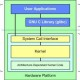Henry - 4 years ago 85
C Question

# Program works in Mac OS, but not in Windows

Please excuse the copy-paste from another site, like this one:

I wrote a program to store data in a table, linked like a linked list (don't know if that's an original idea-- I came up with it myself, but it might not be a new one). It compiles successfully on Windows, but when I run it, it just says,

"dlt_table.exe has encountered an error and needs to close."

The only time I've had this issue was one time when I tried to dereference a null pointer out of morbid curiosity. Realizing that the system simply may have been returning null pointers from
`malloc()`
, I tried checking for the error. Still nothing.

Then, I tried it on my Mac. It built without a problem, and, better yet, the algorithms I came up with actually worked! But I'm still puzzled as to why it didn't work with Windows. Here is the code:

``````#include <stdio.h>
//#include <conio.h> (Windows only- taking it out for Mac dev)
#include <stddef.h>
#include <stdlib.h>

/*############################################################################*/

/*Node structure-- this is what the table is built off.*/
typedef struct dlt_node {
int value;
struct dlt_node *left, *right, *up, *down;
} dlt_node_t;

/*############################################################################*/

/*Function prototypes-- visible at bottom of file.*/
dlt_node_t *make_table(void);
int len_table(dlt_node_t *bucket);
int parse_table(dlt_node_t *bucket);

/*############################################################################*/

main()
{
dlt_node_t *bucket = make_table();

int table_size = len_table(bucket);
printf("Table size: %i\n", table_size);

parse_table(bucket);

//getch();
return 0;
}

/*############################################################################*/

/*Make a table, and return the table's bucket.*/
dlt_node_t *make_table(void)
{
/*Allocate structures.*/
dlt_node_t *bucket = (struct dlt_node*) malloc(sizeof(struct dlt_node));
dlt_node_t *node1 = (struct dlt_node*) malloc(sizeof(struct dlt_node));
dlt_node_t *node2 = (struct dlt_node*) malloc(sizeof(struct dlt_node));
dlt_node_t *node3 = (struct dlt_node*) malloc(sizeof(struct dlt_node));

/*Check for NULL's.*/
if(bucket == NULL || node1 == NULL || node2 == NULL || node3 == NULL){
printf("ERR: ENOMEM.\n");
//             getch();
return;
}

/*Assign values, then pointers to other members of the table*/
bucket->value = 1;
node1->value = 2;
node2->value = 3;
node3->value = 4;

bucket->left = NULL;
bucket->right = node1;
bucket->up = NULL;
bucket->down = node2;

node1->left = bucket;
node1->right = NULL;
node1->up = NULL;
node1->down = node3;

node2->left = NULL;
node2->right = node3;
node2->up = bucket;
node2->down = NULL;

node3->left = node2;
node3->right = NULL;
node3->up = node1;
node3->down = NULL;

/*Return the table's bucket.*/
return bucket;
}

/*Find the number of nodes in the table. Skewed if nodes are randomly deleted.*/
int len_table(dlt_node_t *bucket)
{
dlt_node_t *probe_x, *probe_y;
int x = 0, y = 0;

for(probe_y; probe_y != NULL; probe_y = probe_y->right) y++;

for(probe_x;probe_x!=NULL;probe_x = probe_x->right) x++;

return x*y;
}

/*Parse the table, print values.*/
int parse_table(dlt_node_t *bucket){
dlt_node_t *current, *current_row = bucket;

for ( current_row; current_row!=NULL; current_row = current_row->down ){
current = current_row;
for ( current; current != NULL; current = current->right ){
printf( "Current value: %i\n", current->value );
}
}
}
``````

Sorry about the copy-paste, nobody on Lockergnome.net had any advice, and they recommended asking here.zdan
Answer Source

In your function `len_table` you have not initialized your pointers `probe_x` and `probe_y`. (They are not default initialised to NULL in c). So in the first iteration of your loops, your program is likely to crash.

Recommended from our users: Dynamic Network Monitoring from WhatsUp Gold from IPSwitch. Free Download